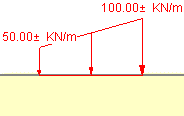Join one of our upcoming courses taking place around the world! Explore now

## Search Results

If your model includes Distributed or Line Loads, then the Load Magnitudes can be treated as Random Variables in a Slide2 Probabilistic Analysis.

If Distributed or Line Loads exist, then you can define the Load Magnitude(s) as Random Variables, as follows.

1. First, you must select the load. The easiest way to do this is by using the Right-Click shortcut. Right-click the mouse anywhere on a load, and a popup context menu will appear. Select Statistical Properties from the popup context menu. You will see the Load Statistics dialog.
2. OR – you can also select a load, by selecting the Loads option from the Statistics menu. Select a load by LEFT clicking on the load. Then right-click and select Done Selection from the popup menu, or you can press the Enter key. You will see the Load Statistics dialog.
3. In the Load Statistics dialog, select the checkbox for the desired Load magnitude(s) that you wish to define as Random Variables.
• If the Load is a Line Load, or a Distributed Load with a Constant magnitude, then only a single magnitude can be defined as a Random Variable.
• If the Load is a Distributed Load with a Triangular distribution, then the magnitude at either end, (or both ends) can be defined as Random Variables.
4. Define the statistical parameters for the selected load magnitude(s). Select a Statistical Distribution, and enter a Standard Deviation (if applicable), and Relative Minimum and Maximum Values.
5. For more information about the statistical parameters which are used to define Random Variables in Slide2, please see the Random Variables topic.
6. Select OK in the Load Statistics dialog.
7. When a Load magnitude is defined as a Random Variable, a "plus / minus" ( ± ) symbol will be displayed on the screen, beside the Load Magnitude, to indicate that the Load Magnitude has been defined as a Random Variable for the Probabilistic Analysis. This is shown in the following figure.Load Magnitudes defined as Random Variables

You cannot define the Load Orientation (Angle) or Location as Random Variables, only the Load Magnitudes can be defined as Random Variables.

## Sensitivity Analysis

If you are performing a Sensitivity Analysis using the Load Magnitude(s), then you must define the Minimum and Maximum values in the Load Statistics dialog, as described above for a Probabilistic Analysis. The Sensitivity Analysis will be carried out by uniformly varying the Load Magnitude(s) between the Minimum and Maximum values, in 50 equal increments.

• If you are performing a Sensitivity Analysis, but you are NOT performing a Probabilistic Analysis, then the Distribution and Standard Deviation options will be disabled in the Load Statistics dialog, since they are not applicable for a Sensitivity Analysis.
• If you are performing BOTH a Sensitivity Analysis AND a Probabilistic Analysis, then the Sensitivity Analysis will use the Minimum and Maximum values you have defined for the load magnitude(s). It will ignore the parameters which are not relevant to the Sensitivity Analysis (i.e. Distribution and Standard Deviation, which are only applicable for the Probabilistic Analysis).